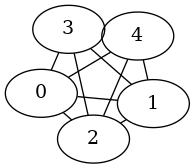# Conversion¶

An example showing how to use the interface to the `pygraphviz.AGraph` class to convert to and from graphviz.```import networkx as nx

G = nx.complete_graph(5)
A = nx.nx_agraph.to_agraph(G)  # convert to a graphviz graph
X1 = nx.nx_agraph.from_agraph(A)  # convert back to networkx (but as Graph)
X2 = nx.Graph(A)  # fancy way to do conversion
G1 = nx.Graph(X1)  # now make it a Graph

A.write("k5.dot")  # write to dot file